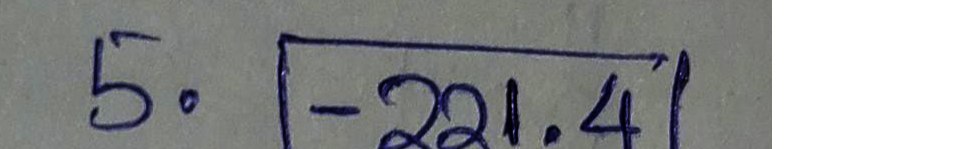Question

Reaction Engineering

5.Given that the enthalpy of combustion of propanal, C3H,0(1) is -1816.5kJ/mol when liquid water is formed, what is the molar enthalpy of formation of propanal?Verified### Question 45643Reaction Engineering

\text { Consider a feedback control system with P-controller, } G_{v}=\frac{0.5}{25+1}, G_{P}=
\frac{2}{35+1}, G_{m}=0.2 . \text { Find the range of the controller gain } K_{c} \text { to ensure the stability }
following Routh stability method.

### Question 45642Reaction Engineering

The Exothermic reaction A → 2B is taking place in the CSTR reactor shown inFig. E.9. Component A is considered as expensive material. Since the reaction isexothermic the temperature of the tank need to be controlled at 60 °C to avoidtemperature run-away. Also, the outlet concentration of CA is required to be atset-point CP. The level of the CSTR product mixture is controlled to avoid theoverflow.
1. What is the type of valves V-1, V-2, V-4?
2. What is the type of controllers used for the control loops in the process?
3. What is the action of the controller selected (reverse, direct)?
4. Draw a P&ID diagram of the schematic given for each control loops.

### Question 45641Reaction Engineering

The blending process shown in Fig. E.8 is used to dilute a toxic material in stream1 with pure water fed to the tank as stream 2. Heater is implemented in the process since the mixing is endothermic. The mass fraction of the outlet toxic component-need to be maintain at specific value (xSP0.5). Also, the temperature need to be between 75 – 80 °C to achieve proper mixing. Answer the following questions:
1. What is the type of valves V-1, V-2?
2. What is the type of controllers used for both control loops in the process?
3. What is the action of the controller selected (reverse, direct)?
4. Draw a P&ID diagram of the schematic given for both control loops.

### Question 45640Reaction Engineering

The outlet concentration of CSTR reactor is related to the inlet temperature of thereactor by:
\frac{C_{A}(S)}{T_{i}(S)}=\frac{K}{\tau^{2} S^{2}+2 \tau \zeta S+1}
When the inlet temperature is suddenly changed from 40 °C to 41 °C. The outletconcertation response is shown in in Fig. E.7. Determine the following:
a) The Steady-state gain?
b) value of overshoot and decay ratio?
c) The Damping coefficient?
d) The value of the time constant?

### Question 45639Reaction Engineering

The outlet concentration of CSTR reactor is related to the inlet flow of the reactorby:
\frac{C_{A}(S)}{Q_{i}(S)}=\frac{3}{\tau^{2} S^{2}+2 \tau \zeta S+1}
When the inlet flow is suddenly changed from 2L/s to 4L/s. The outlet concertation showed an oscillatory response. The outlet concentration reached a maximum value of 10 mol/L. knowing that the outlet concentration has a value of 2 mol/L before the sudden change. Also, the time recorded between two successive peak was 3 minutes. Determine the following:
a) The value of overshoot and decay ratio?
b) The Damping coefficient?
c) The peak time?
d) The value of the time constant?

### Question 45638Reaction Engineering

Consider the following storage tank shown in Fig. E.5. The volumetric flow rateare varied with time. The valve used on the outlet flow is linear with resistanceR1 = 0.3. The cross-sectional area of the tank is 3 m?. The height of the liquid inthe tank at steady-state condition h = 4 m. Answer the following:
\text { a) Obtain a transfer function relating liquid level with inlet flow rate } \frac{H^{\prime}(S)}{Q_{1}^{\prime}(S)}
b) For a step-change in the inlet flow from 3m³/s to 4m³/s, find the final value of the height of the liquid in the tank.

### Question 45637Reaction Engineering

Solve the following ODE using Laplace method:
\frac{d^{2} x}{d t^{2}}+6 \frac{d x}{d t}+4 x=3 e^{-t}, \frac{d x}{d t}(0)=x(0)=0

### Question 45636Reaction Engineering

A completely enclosed stirred-tank heating process is used to heat an incomingstream whose flow rate varies. The heating rate from this coil and the volume areboth constant. Develop a mathematical model for the process if the heat losses tothe atmosphere occur.
1. p and Cpare constants.
2. U, the overall heat transfer coefficient, is constant.
3. A, is the surface area for heat losses to ambient.
4. T; > Ta (inlet temperature is higher than ambient temperature).

### Question 45635Reaction Engineering

A closed stirred-tank reactor with two compartments is shown in Fig. E2.6. The basic idea is to feed the reactants continuously into the first compartment, where they will be pre-heated by energy liberated in the 2nd order exothermic reaction2A→B, which is anticipated to occur primarily in the second compartment. The wall separating the two compartments is quite thin, thus allowing heat transfer;the outside of the reactor is well insulated; and a cooling coil is built into the second compartment to remove excess energy liberated in the reaction. Develop a mathematical model for this process.

### Question 45634Reaction Engineering

Two tanks are connected together in the following unusual way in Fig. E2.3. Develop a model for this system that can be used to find hj, h2,W2, and w3 as functions of time for any given variations in inputs.
1. The density of the incoming liquid,p, is constant.
2. The cross-sectional areas of the two tanks are A1 and A2.
3. w2 is positive for flow from Tank 1 to Tank 2.
4. The two valves are linear with resistances R2 and R3.

### Submit query

Getting answers to your urgent problems is simple. Submit your query in the given box and get answers Instantly.

### Submit a new QuerySuccess

Assignment is successfully created